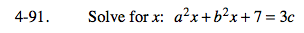### Home > PC > Chapter 4 > Lesson 4.2.2 > Problem4-91

4-91.Isolate the x on the left side by subtracting 7 from both sides.

Factor out an 'x' on the left side.

Divide both sides by x's factor solving for x.

a2x + b2x = 3c − 7

x(a2 + b2) = 3c − 7

$x=\frac{3c-7}{(a^2+b^2)}$